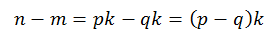# Reducing Fractions

by Zoran Horvat

## Problem Statement

Given two integers n >= 0 and m > 0, write a function which reduces fraction n/m by producing a pair of relatively prime numbers p and q such that n/m=p/q.

Example: Suppose that initial fraction is 247/26. Reduced fraction is 19/2.

## Problem Analysis

To reduce a fraction, we need to find a number which divides without remainder both the numerator and the denominator. Larger the divisor, smaller the numerator and the denominator will be after being divided by it. The largest divisor for two numbers is called greatest common divisor (or greatest common factor). When two numbers are divided by their GCD, they become relatively prime. This means that there is no common factor for the two numbers anymore.

So the problem of reducing a fraction is to calculate greatest common divisor of its numerator and denominator k, such that n=pk and m=qk. After dividing both numbers in fraction n/m with k, we obtain the reduced fraction p/q.

Now we will focus on the problem of calculating GCD for two integer numbers n and m. First of all, notice that GCD(n, m) is the same as GCD(m, n). Consequently, we are free to assume that n >= m (if it is not, then we can simply swap the values). Next, we will assume that there is some value k >= 1 such that it divides both n and m: n=pk, m=qk. Finally, we will notice that if k divides n and m, then k also divides their difference n-m. This is proven easily:We can follow the same logic and claim that k also divides n-2m, n-3m, ..., n-am. This process ends with value a such that n-am becomes smaller than m. In other words, n-am is the remainder after dividing n by m:By combing this equation with relations to GCD we can prove what we have already predicted: that remainder when dividing n by m shares the same GCD with original numbers n and m:Since n mod m is less than both n and m, it seems that we have transformed the original problem into a smaller one which yields the same result. We are free to repeat the step and to produce another pair of even smaller numbers. The question is when does this process end? Well, at some point the modulo will eventually have to become zero. At that point we will observe that GCD(x, 0) = x for any positive integer x. This gives us the ending criterion: the process of replacing the lower argument to GCD with modulo result ends when modulo becomes zero. At that moment the other argument, which is still positive, becomes the overall result, i.e. it is the requested GCD(n, m).

Here is the simple recursive solution to the problem:

``````int gcdRecursive(n, m)
{

if (n < m)
return gcdRecursive(m, n);

if (m == 0)
return n;

return gcdRecursive(m, n % m);

}
``````

The first if statement ensures that first argument is greater or equal to the second argument, which is prerequisite to calculate the first modulo. Second if statement tests the ending criterion: if smaller argument has reached zero, then the first argument is overall result. Finally, if both arguments are positive, with first argument being greater or equal to the second argument, then we repeat the calculation on modified arguments: smaller number becomes the first argument, and result of modulo operation becomes the second argument.

Function presented above is quite handy, but needlessly complicated in terms of recursive calls. It can be easily transformed into iterative form:

``````int gcd(n, m)
{

if (n < m)
{
int tmp = n;
n = m;
m = tmp;
}

while (m > 0)
{
int tmp = n % m;
n = m;
m = tmp;
}

return n;

}
``````

This implementation produces the same output as the recursive one. This function can then be used to reduce the fraction:

``````void reduceFraction(n in/out, m in/out)
{
int k = gcd(n, m);
n /= k;
m /= k;
}
``````

## Implementation

Below is the complete implementation of console application in C# which inputs fractions, reduces them and prints them out reduced.

``````using System;

namespace ReducingFractions
{

public class Program
{

static int Gcd(int n, int m)
{

if (n < m)
{
int tmp = n;
n = m;
m = tmp;
}

while (m > 0)
{
int tmp = n % m;
n = m;
m = tmp;
}

return n;

}

static void ReduceFraction(ref int n, ref int m)
{
int k = Gcd(n, m);
n = k;
m = k;
}

static void Main(string[] args)
{

while (true)
{

Console.Write("Enter numerator (negative to exit): ");

if (n < 0)
break;

Console.Write("Enter denominator: ");

int p = n;
int q = m;
ReduceFraction(ref p, ref q);

Console.WriteLine("{0} / {1} = {2} / {3}", n, m, p, q);
Console.WriteLine();

}

}

}
}
``````

## Demonstration

When application is run, it produces output like this:

```            ```
Enter numerator (negative to exit): 12
Enter denominator: 16
12 / 16 = 3 / 4

Enter numerator (negative to exit): 3
Enter denominator: 5
3 / 5 = 3 / 5

Enter numerator (negative to exit): 247
Enter denominator: 26
247 / 26 = 19 / 2

Enter numerator (negative to exit): 0
Enter denominator: 9
0 / 9 = 0 / 1

Enter numerator (negative to exit): -1
```
```